## 13.4 Box plots

A boxplot is a convenient way to describe the distribution of the data.

• A simple boxplot:
``````# Create a matrix of 1000 random values from the normal distribution (4 columns, 250 rows)
mat1000 <- matrix(rnorm(1000),
ncol=4)

# Basic boxplot
boxplot(x=mat1000)``````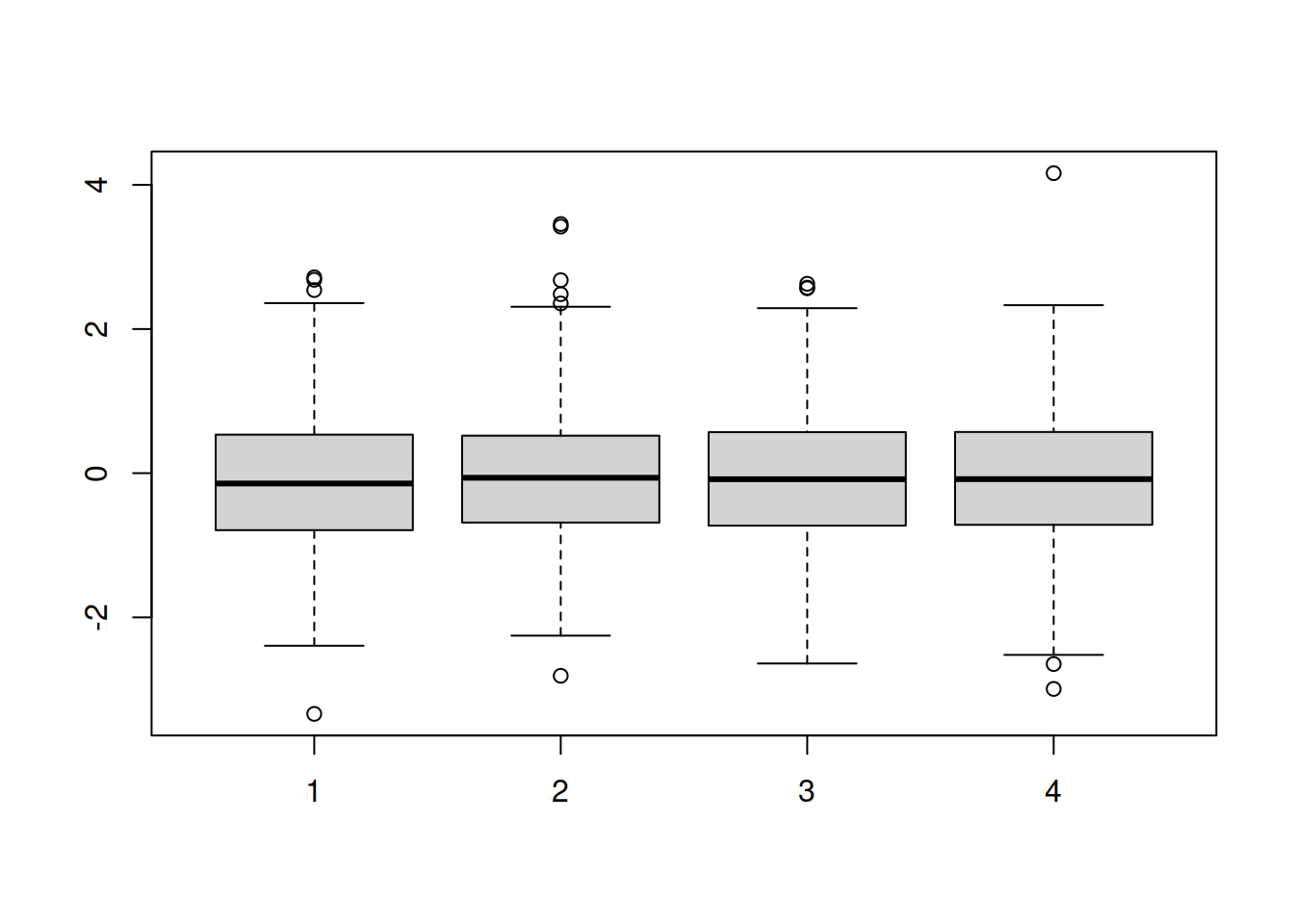• xlab: x-axis label
• ylab: y-axis label
• at: position of each box along the x-axis: here we skip position 3 to allow more space between boxes 1/2 and 3/4
``````boxplot(x=mat1000,
xlab="sample",
ylab="expression",
at=c(1, 2, 4, 5))``````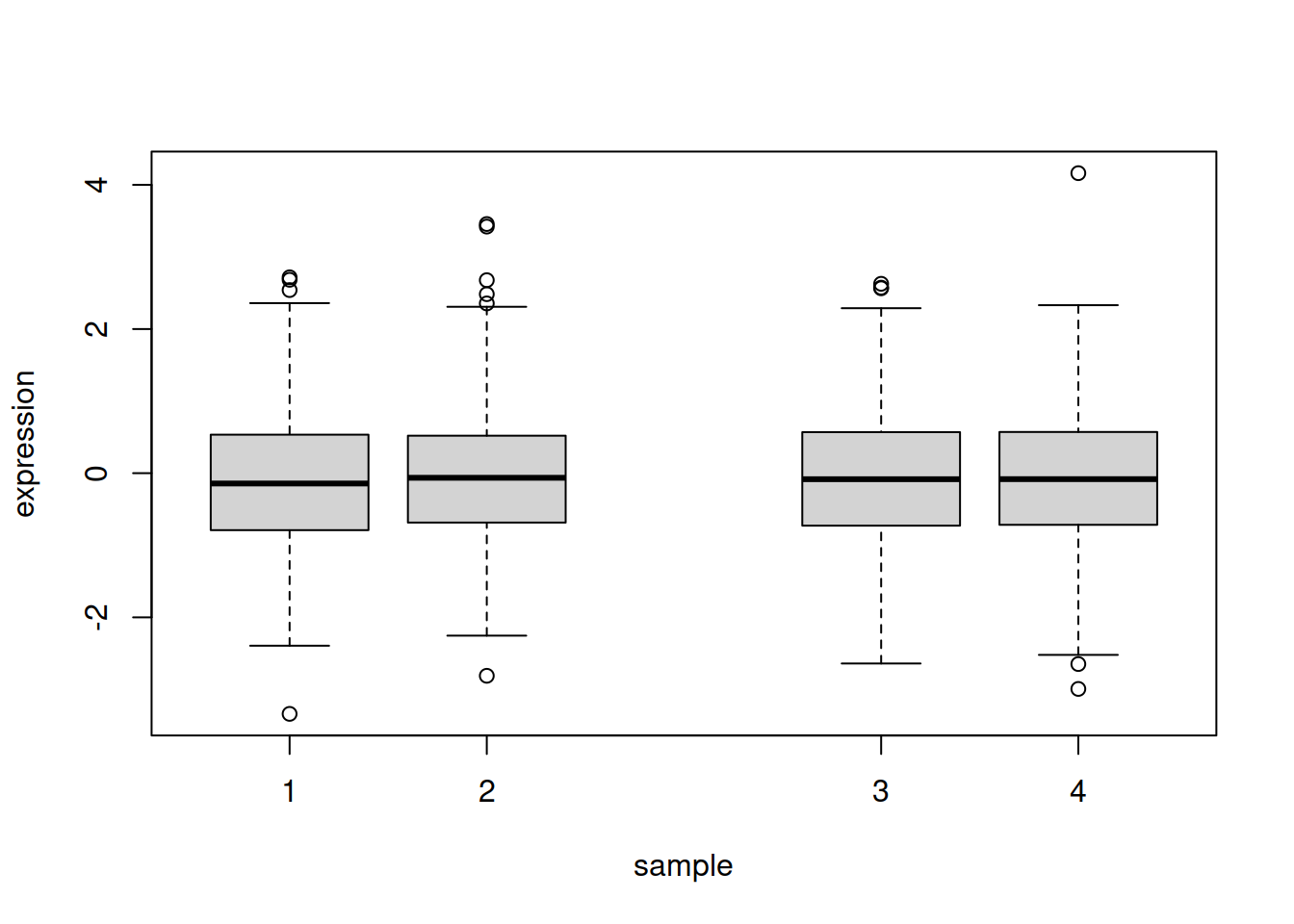• Add an horizontal line at y=0 with abline(); arguments of abline :
• h : y-axis starting point of horizontal line (v for a vertical line)
• col : color
• lwd : line thickness
• lty : line type

NOTE: you can create a vertical line with `abline(v=...)` (v insteald of h)

``````# First plot the box plot as before:
boxplot(x=mat1000,
xlab="sample",
ylab="expression",
at=c(1, 2, 4, 5),
main="my boxplot")

# Then run the abline function
abline(h=0, col="red", lwd=3, lty="dotdash")``````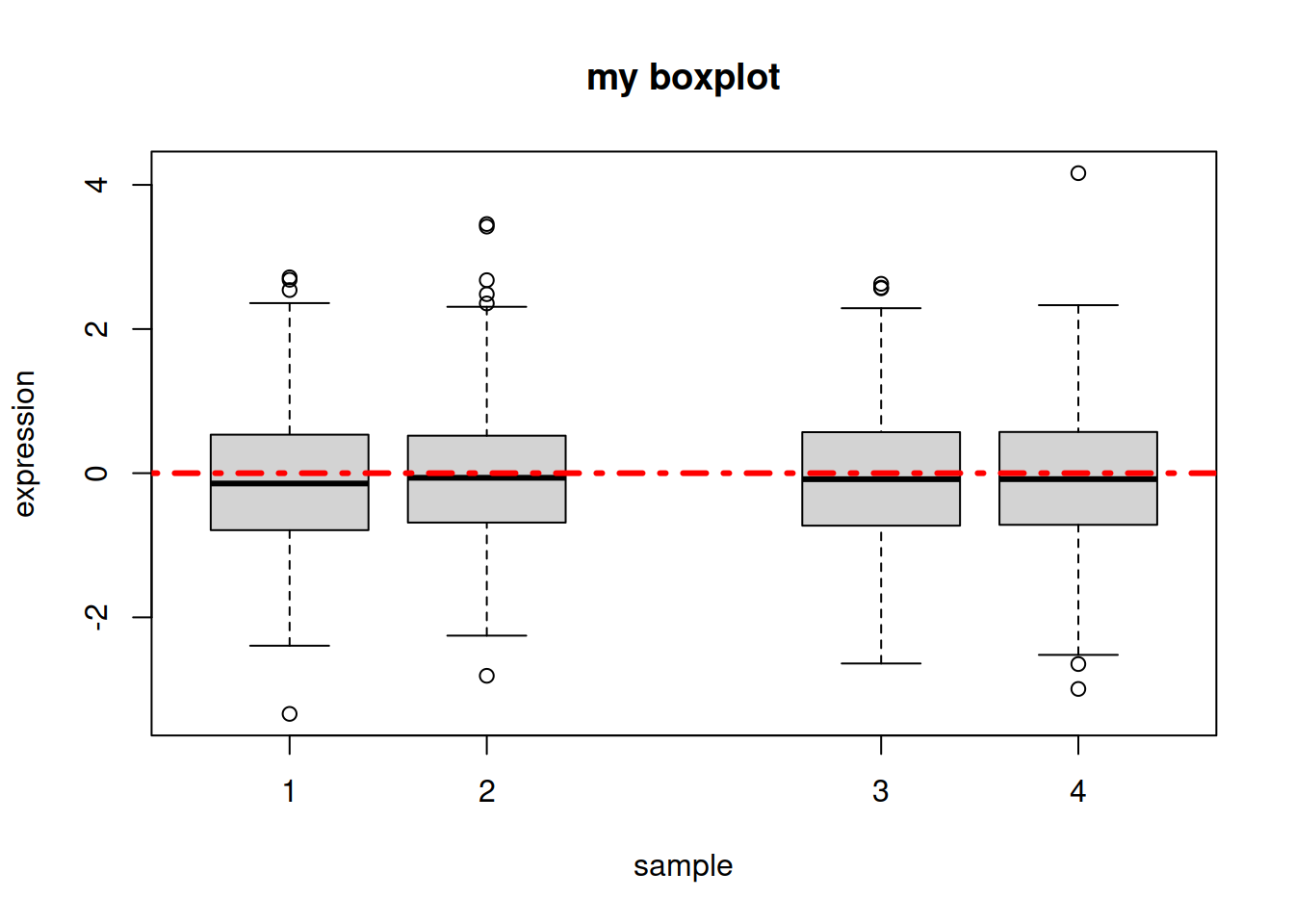• Line types in R: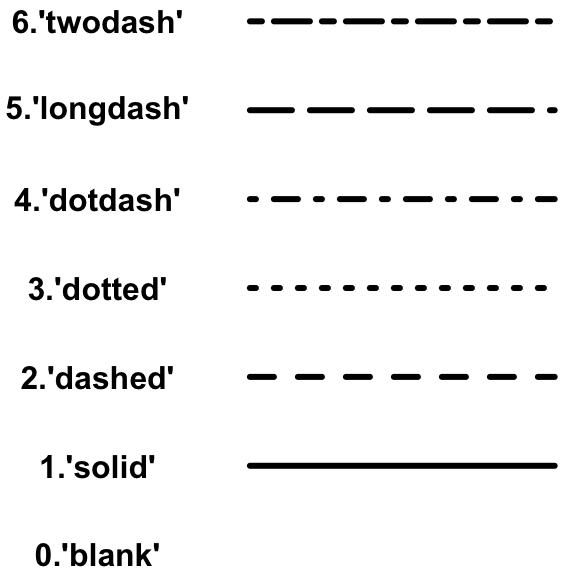• We can also create a boxplot that plots a variable against another variable. For example, going back to our Loblolly data frame, we can create a boxplot of the height (y-axis) for each age (x-axis): one box per age group. Instead of setting parameter x we set parameter formula, as follows:
``boxplot(formula=Loblolly\$height ~ Loblolly\$age)``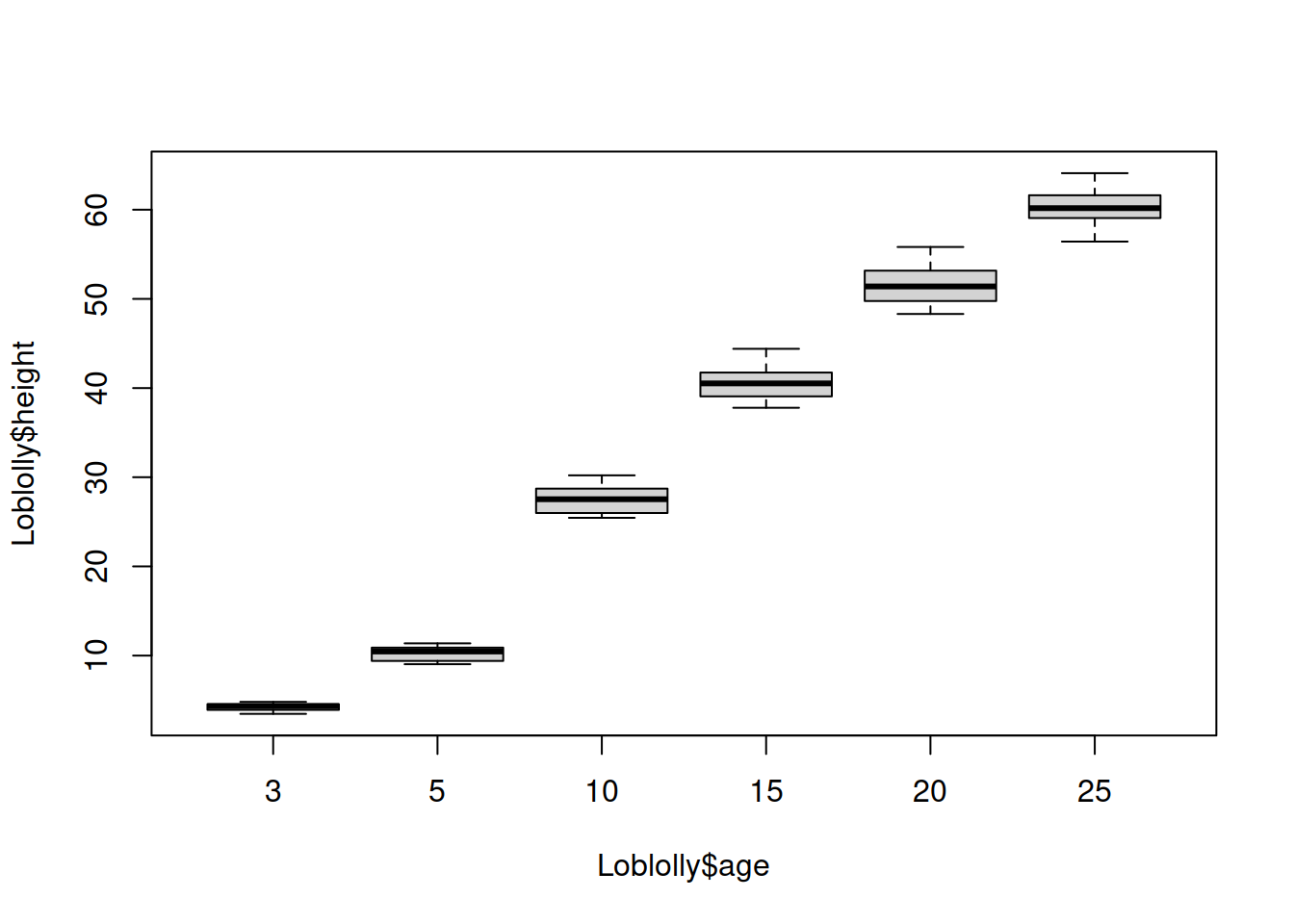HANDS-ON

Let’s go back to our chickwts dataset:

• Create a boxplot that represents the chicken weight for each type of feed supplement.
• Create again the boxplot, but without the sunflower and casein types of feed supplement (you can create a new data frame called chickwts2).
• NOTE: you still see the groups you removed (while there is no data -> no boxes): this is because column `feed` is made of factors. Factors retain the original levels (groups) even when no data is left for those groups. You can run: `chickwts2\$feed <- droplevels(chickwts2\$feed)` to “drop” the levels that do not have values left, and plot again.
• Change the boxes’ colors.
• Add a legend on the top-left corner of the plot, and remove the x-axis labels.
``````# boxplot of weight / feed supplement
boxplot(chickwts\$weight ~ chickwts\$feed)
# remove sunflower and casein
chickwts2 <- chickwts[chickwts\$feed != "sunflower" & chickwts\$feed != "casein", ]
boxplot(chickwts2\$weight ~ chickwts2\$feed)
# drop "levels" from column "feed" containing factors
chickwts2\$feed <- droplevels(chickwts2\$feed)
# plot again after dropping the levels
boxplot(chickwts2\$weight ~ chickwts2\$feed)
# change colors: create a vector
boxcols <- c("lightgreen", "purple", "maroon", "lightblue")
# boxplot with colors (xaxt will remove the x-axis information)
boxplot(chickwts2\$weight ~ chickwts2\$feed,
col=boxcols, xaxt="n")